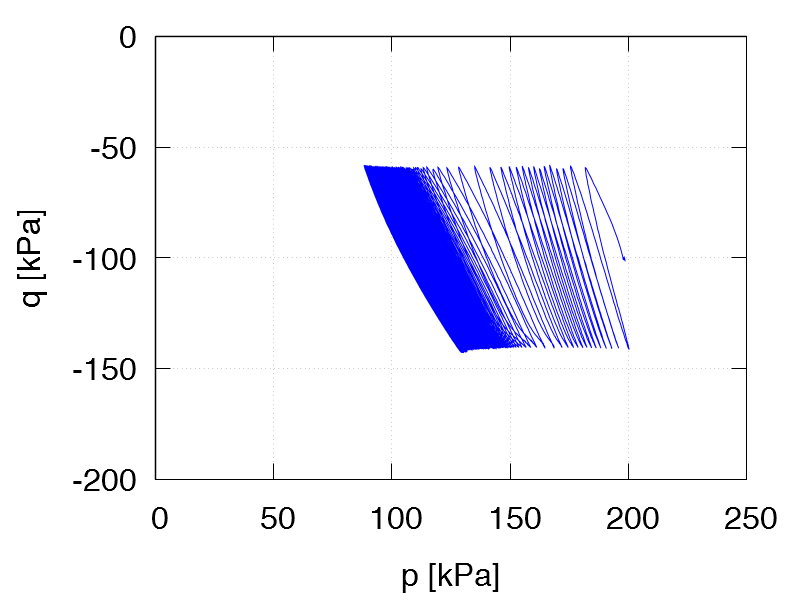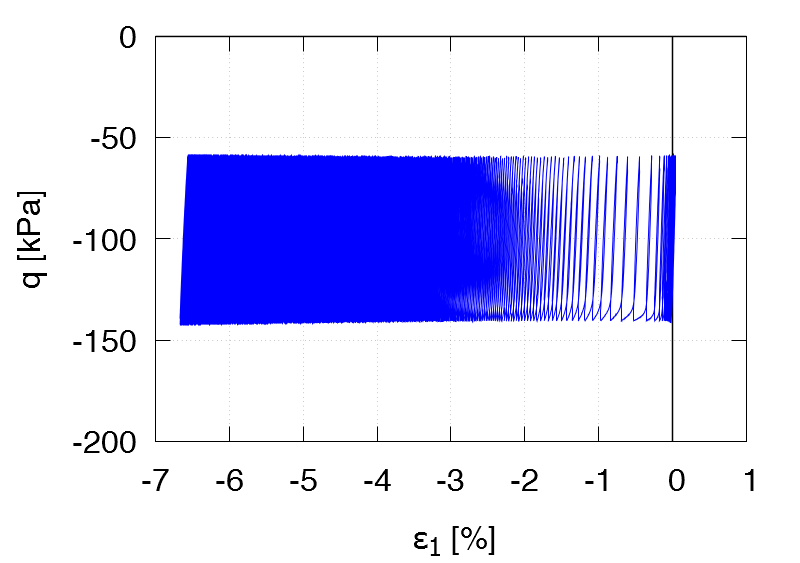Undrained cyclic tests with anisotropic consolidation and stress cycles:   Data of all tests of this series
 TCUA1 ID0 = 0.29 p0 = 300 kPa η0 = 0.5 qampl = 120 kPa Test data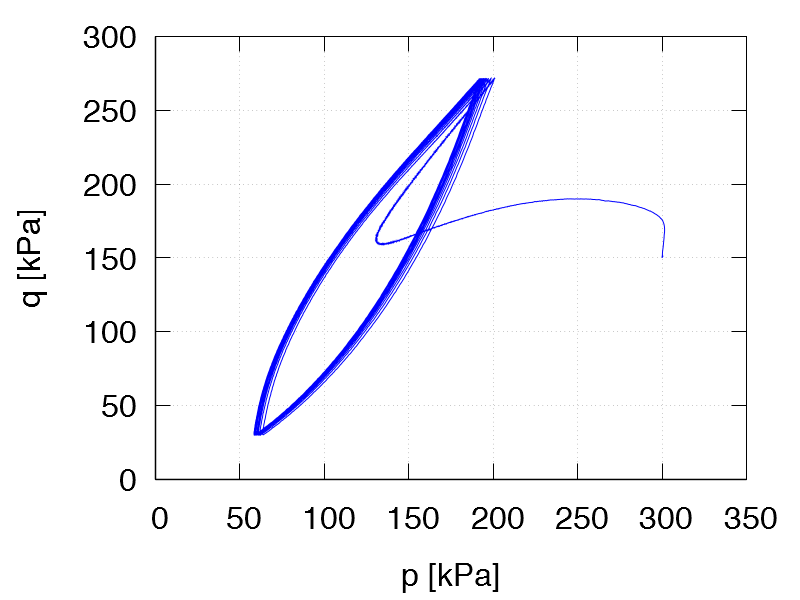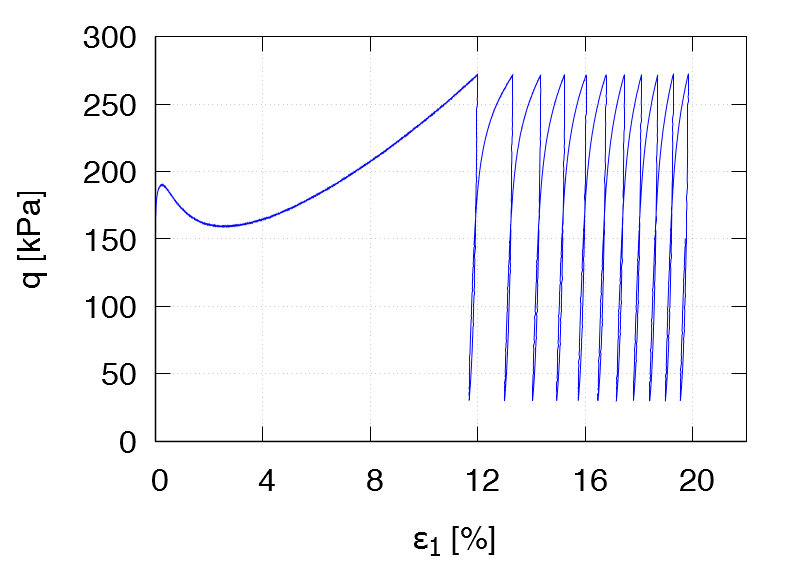TCUA2 ID0 = 0.64 p0 = 300 kPa η0 = 0.5 qampl = 120 kPa Test data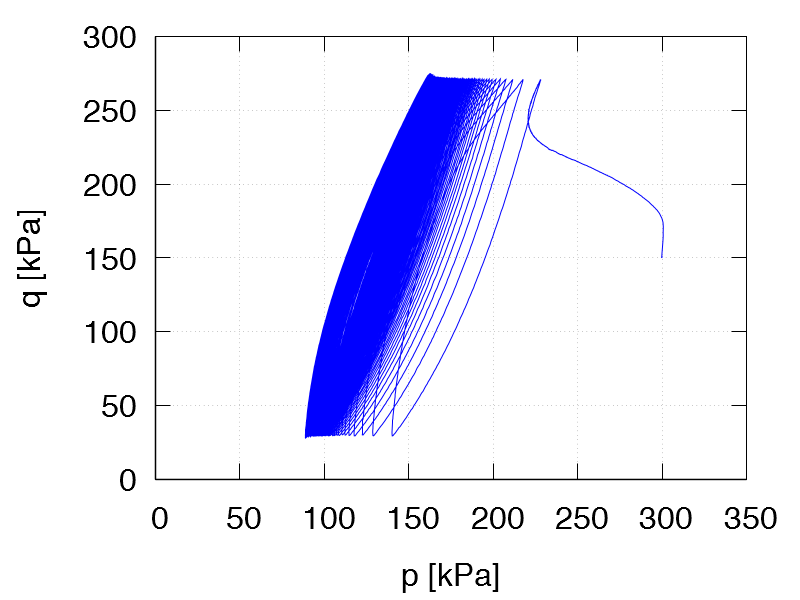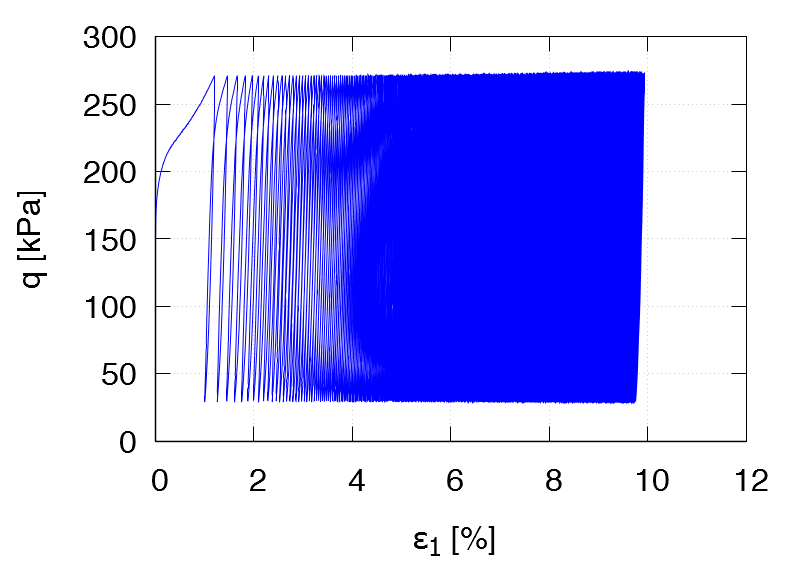TCUA3 ID0 = 0.87 p0 = 300 kPa η0 = 0.5 qampl = 120 kPa Test data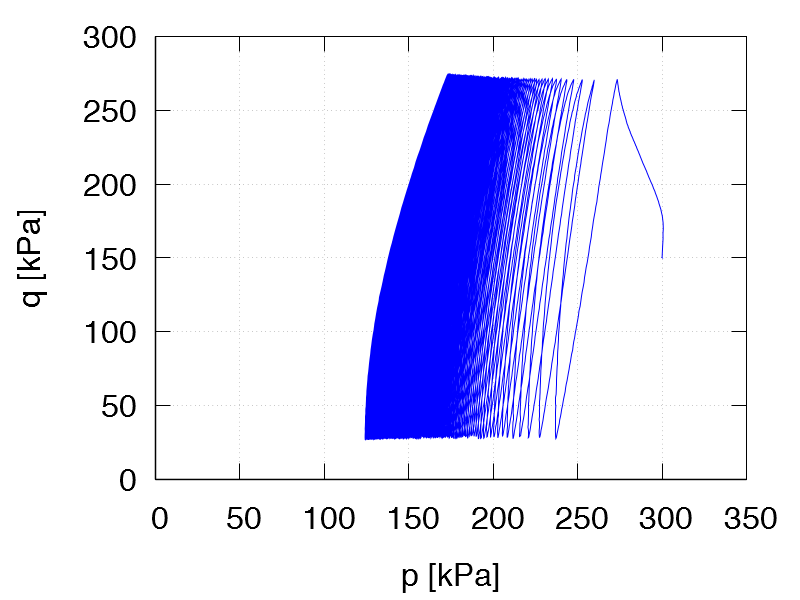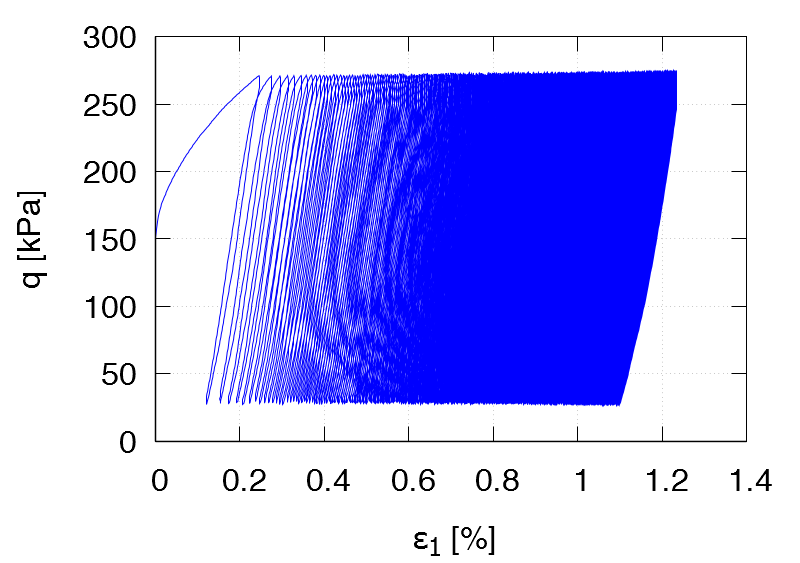TCUA4 ID0 = 0.63 p0 = 300 kPa η0 = 0.5 qampl = 60 kPa 1st drained cycle Test data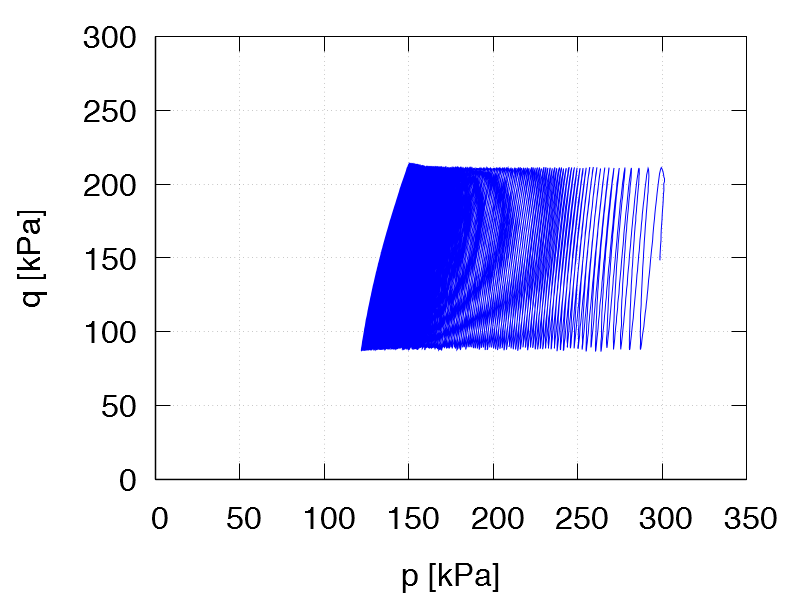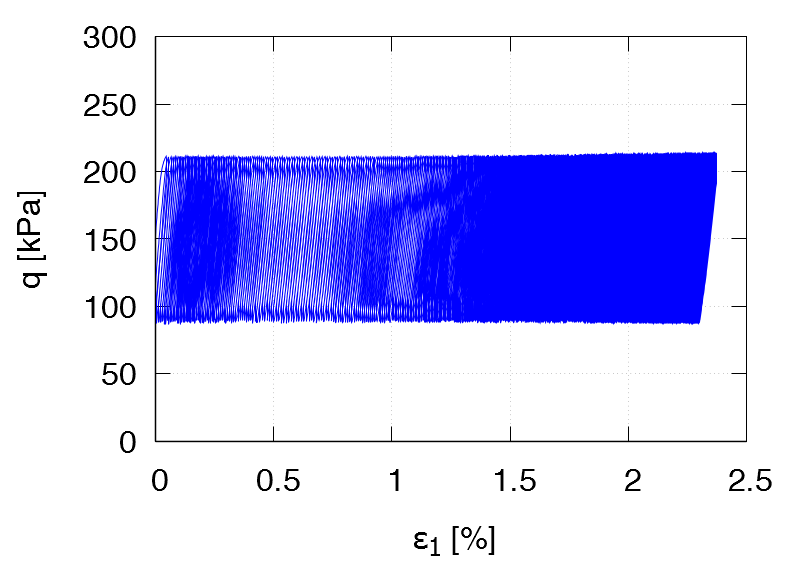TCUA5 ID0 = 0.63 p0 = 300 kPa η0 = 0.5 qampl = 90 kPa 1st drained cycle Test data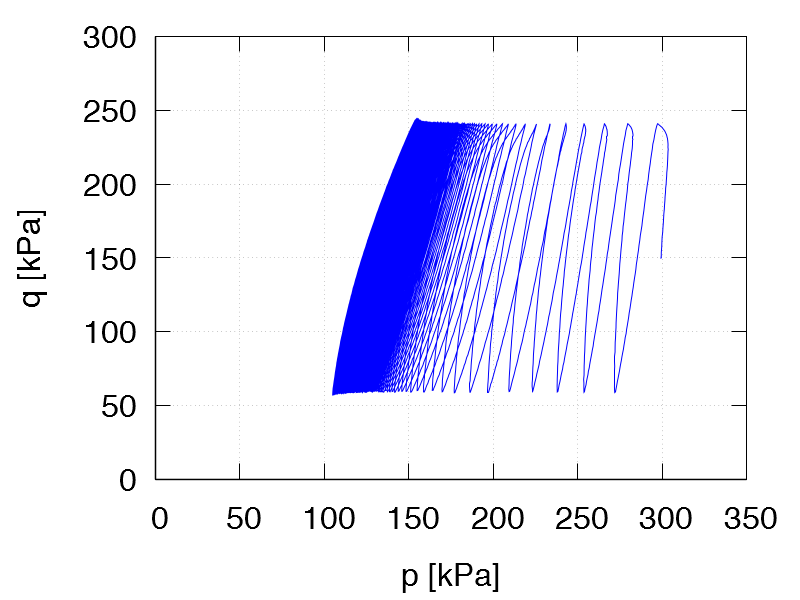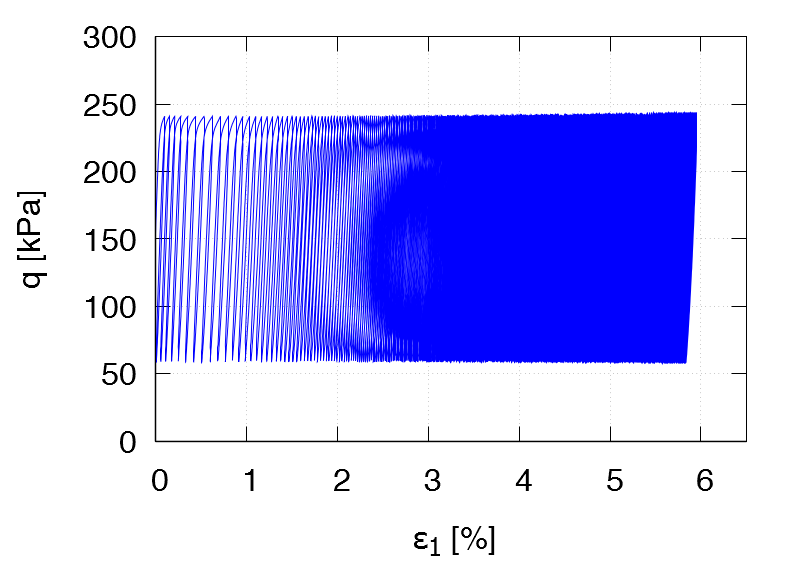TCUA6 ID0 = 0.61 p0 = 300 kPa η0 = 0.5 qampl = 120 kPa 1st drained cycle Test data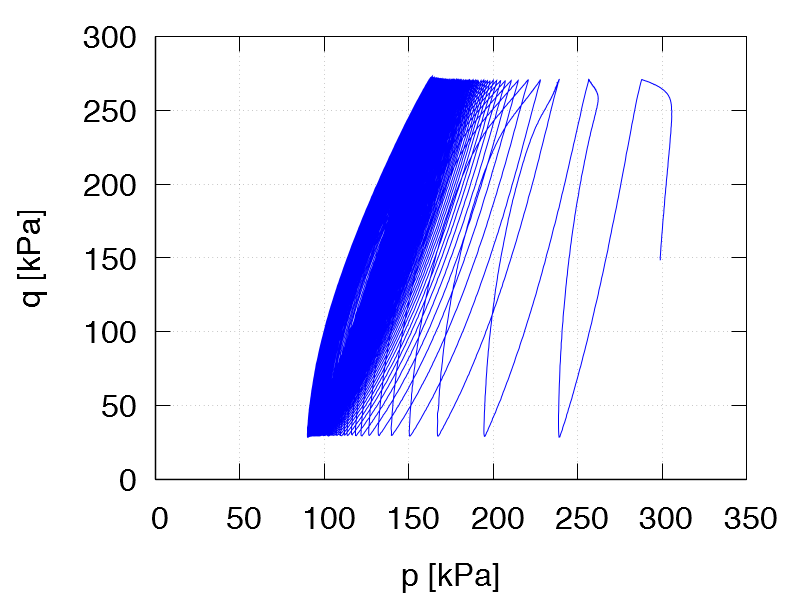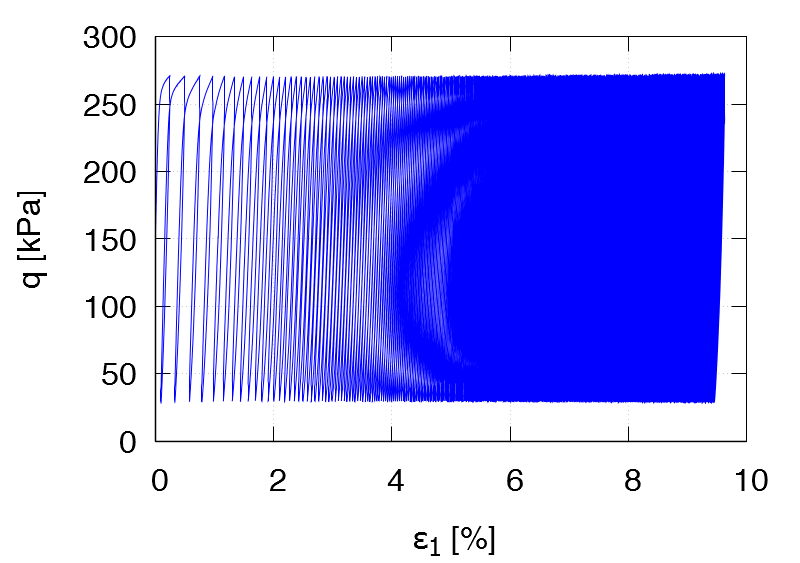TCUA7 ID0 = 0.38 p0 = 300 kPa η0 = 0.5 qampl = 120 kPa 1st drained cycle Test data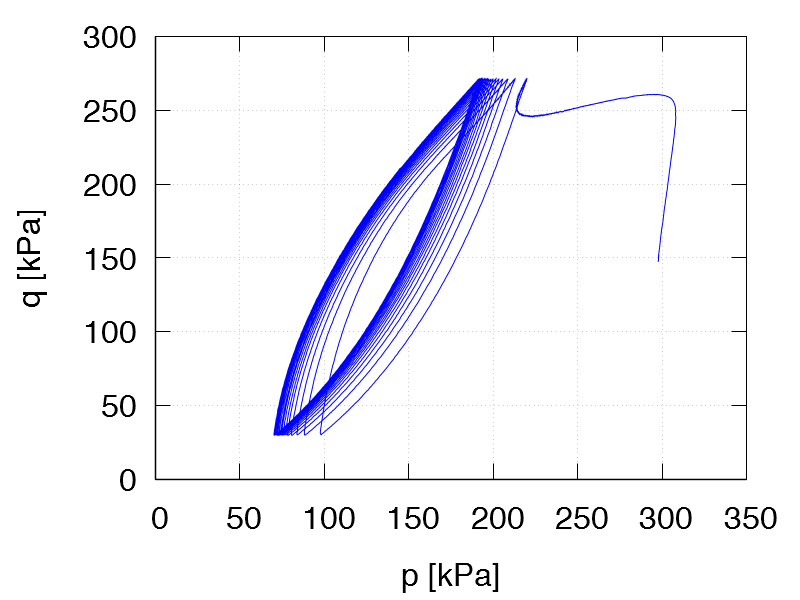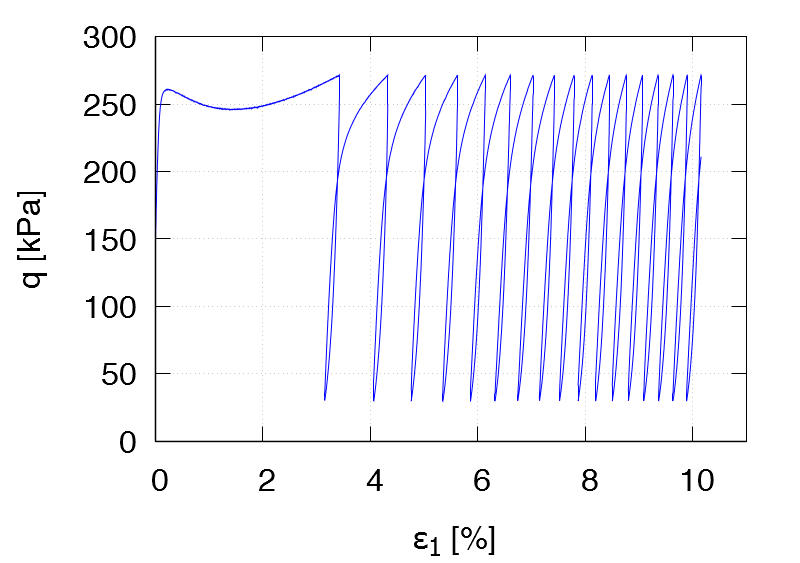TCUA8 ID0 = 0.86 p0 = 300 kPa η0 = 0.5 qampl = 120 kPa 1st drained cycle Test data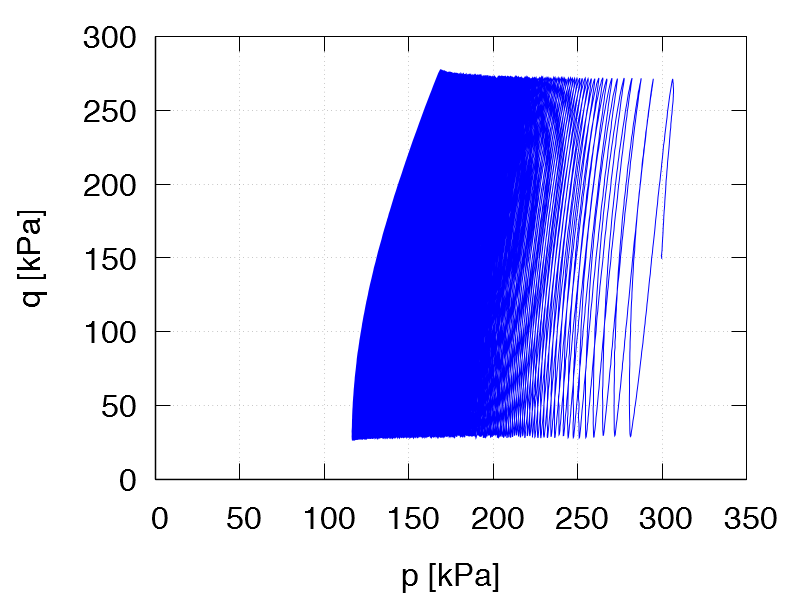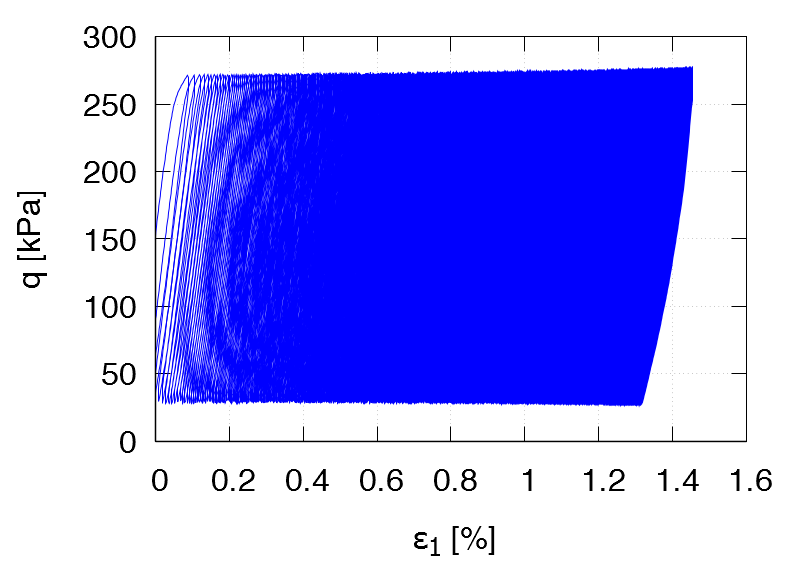TCUA9 ID0 = 0.57 p0 = 100 kPa η0 = 0.75 qampl = 25 kPa 1st drained cycle Test data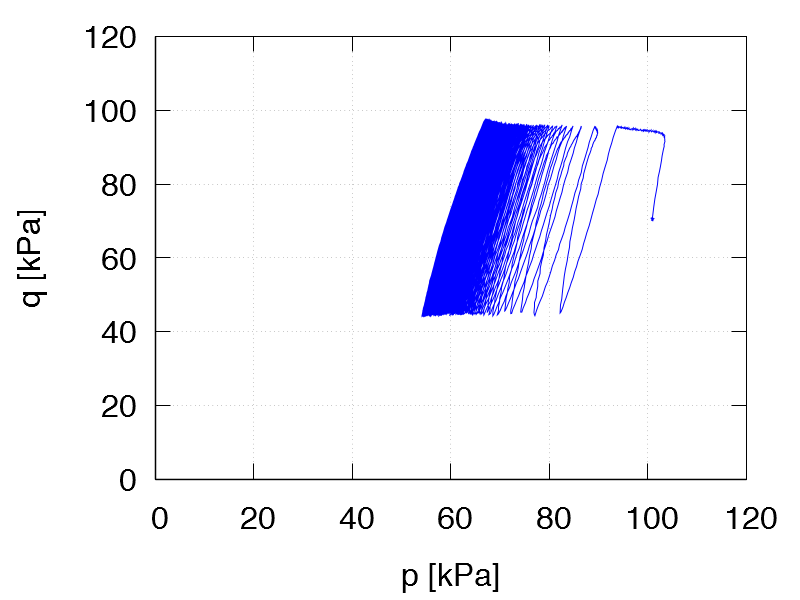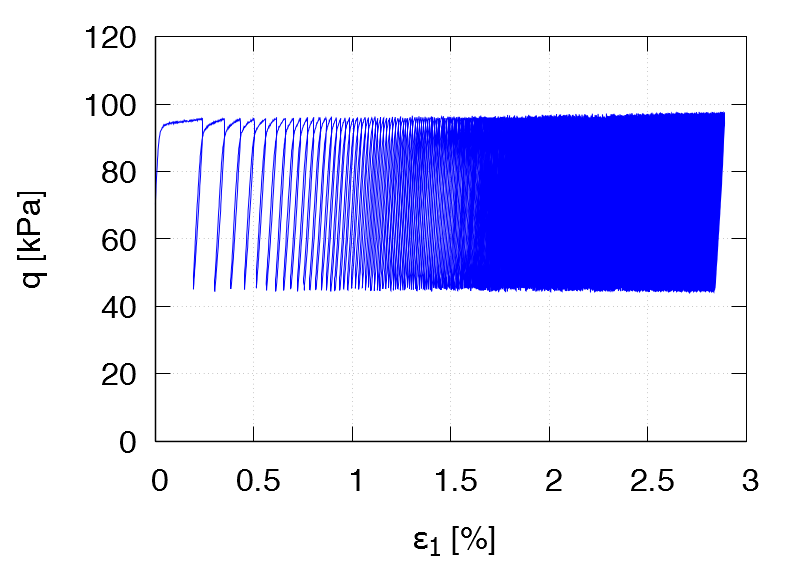TCUA10 ID0 = 0.66 p0 = 200 kPa η0 = 0.75 qampl = 50 kPa 1st drained cycle Test data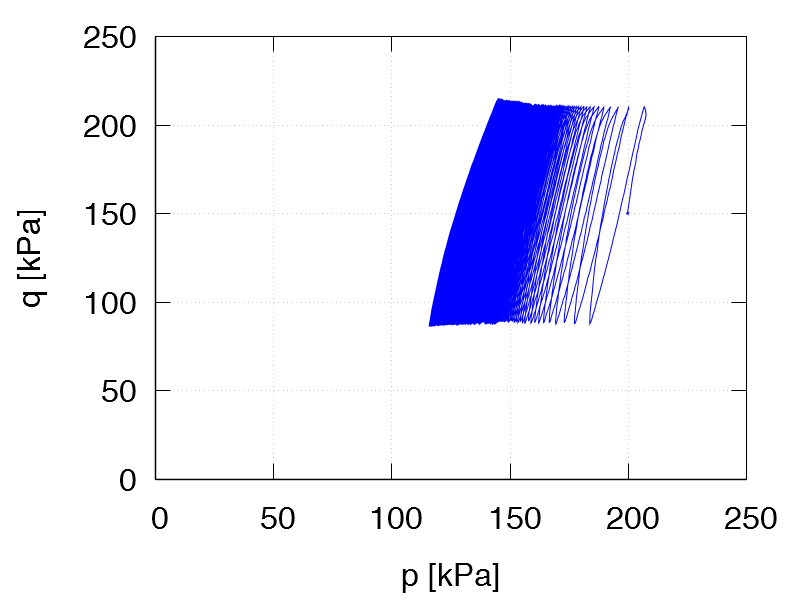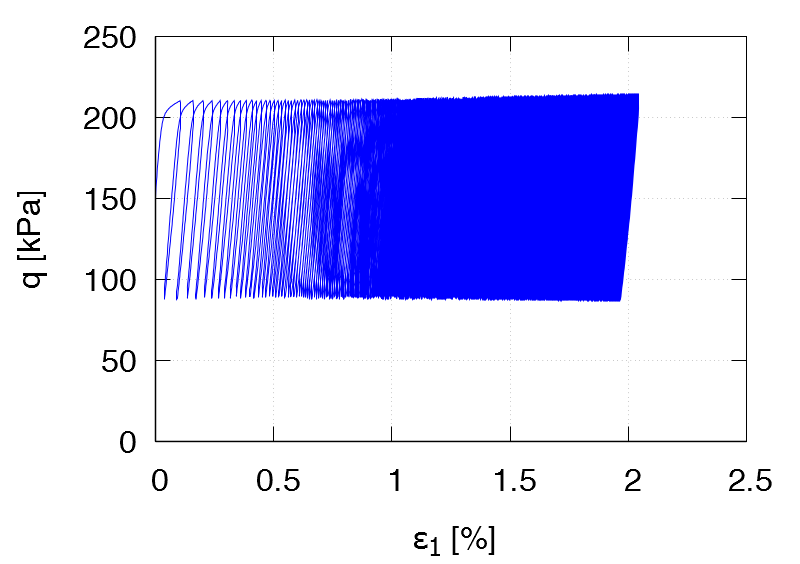TCUA11 ID0 = 0.63 p0 = 300 kPa η0 = 0.75 qampl = 75 kPa 1st drained cycle Test data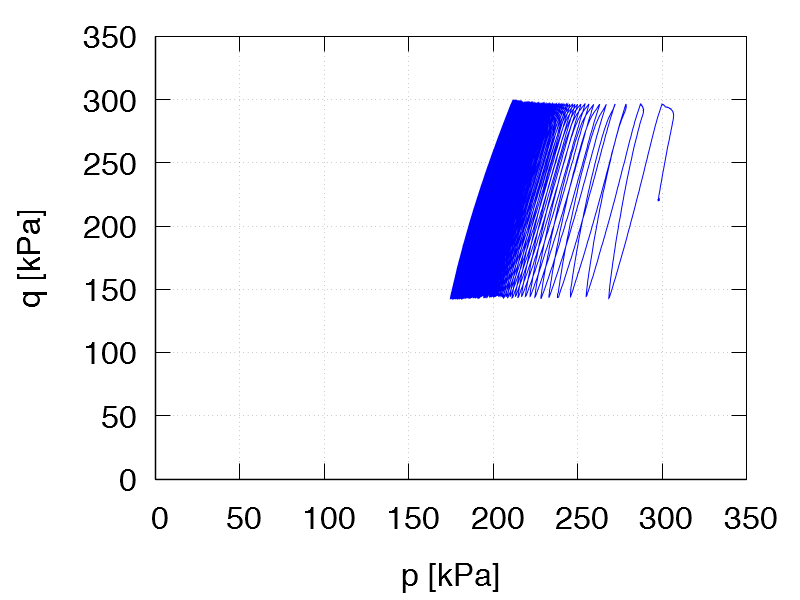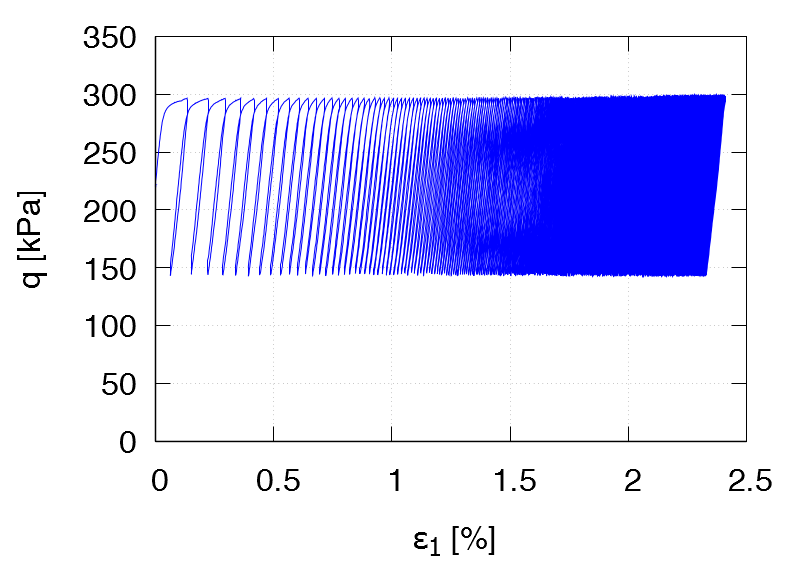TCUA12 ID0 = 0.60 p0 = 200 kPa η0 = 1.0 qampl = 60 kPa 1st drained cycle Test data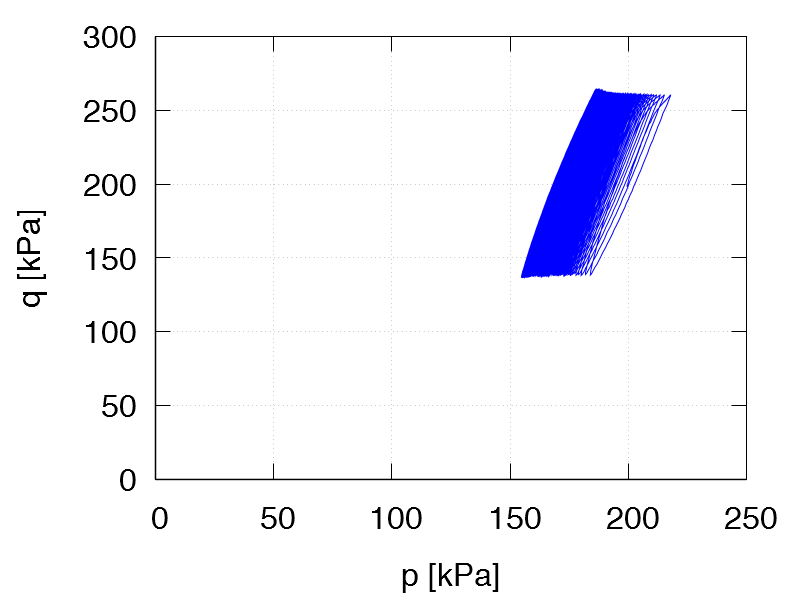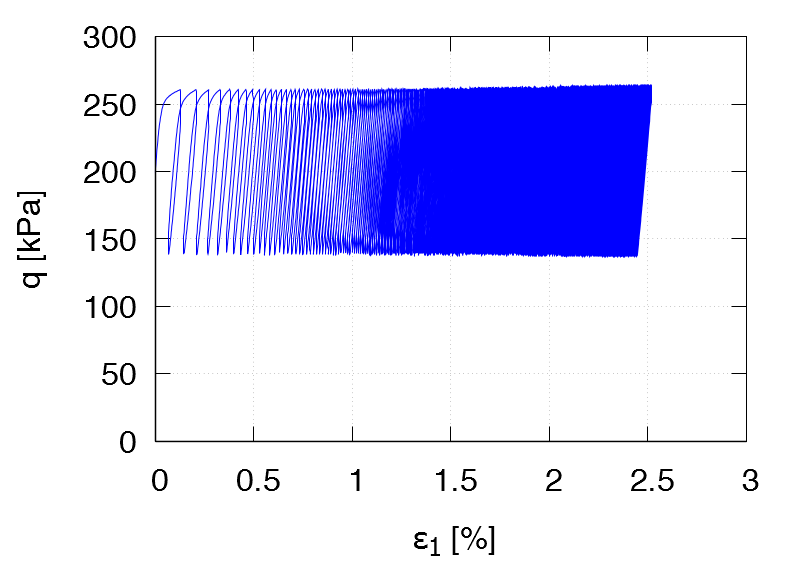TCUA13 ID0 = 0.64 p0 = 200 kPa η0 = 0.75 qampl = 60 kPa 1st drained cycle Test data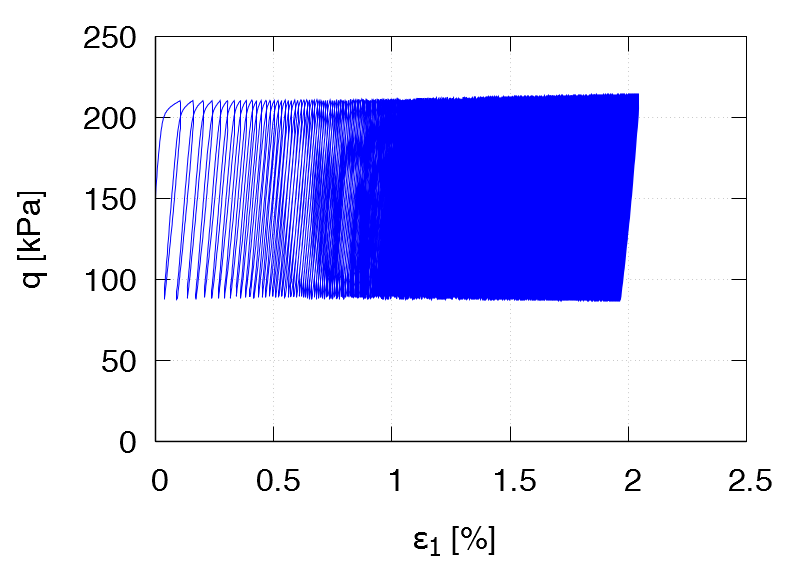TCUA14 ID0 = 0.57 p0 = 200 kPa η0 = 0.5 qampl = 60 kPa 1st drained cycle Test data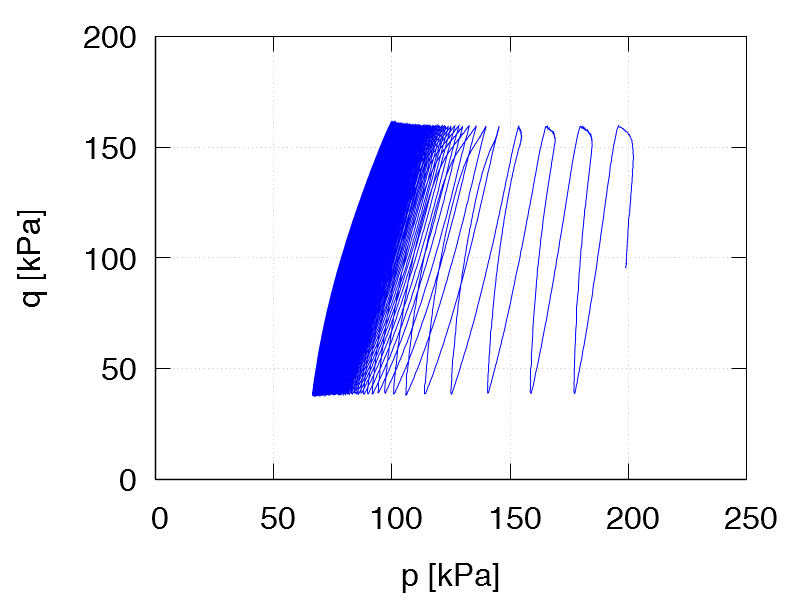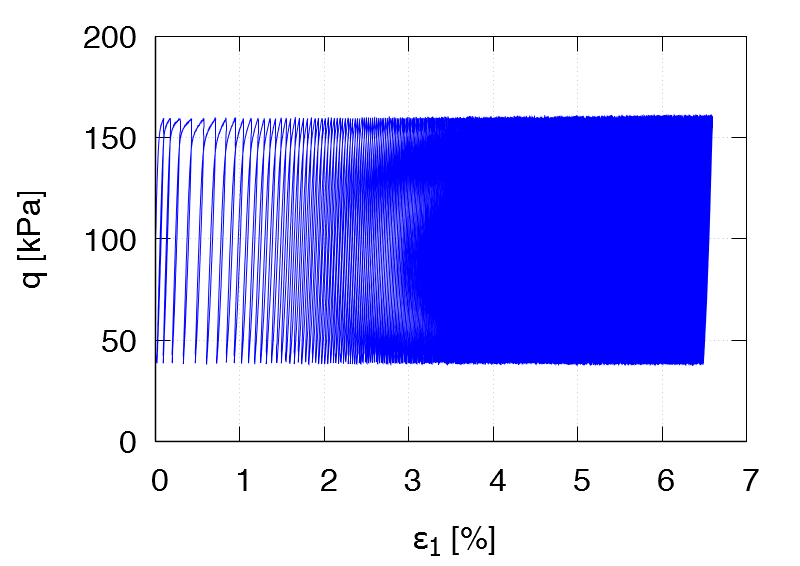TCUA15 ID0 = 0.66 p0 = 200 kPa η0 = 0.3 qampl = 60 kPa 1st drained cycle Test data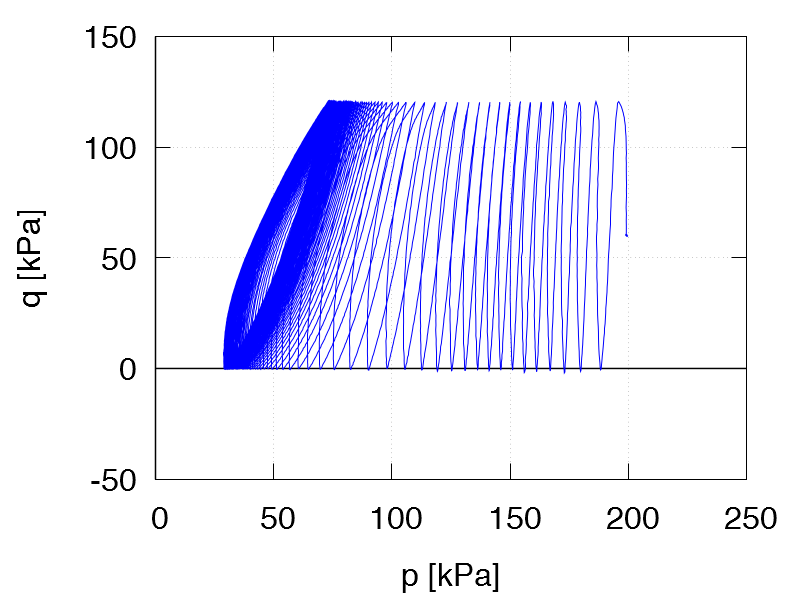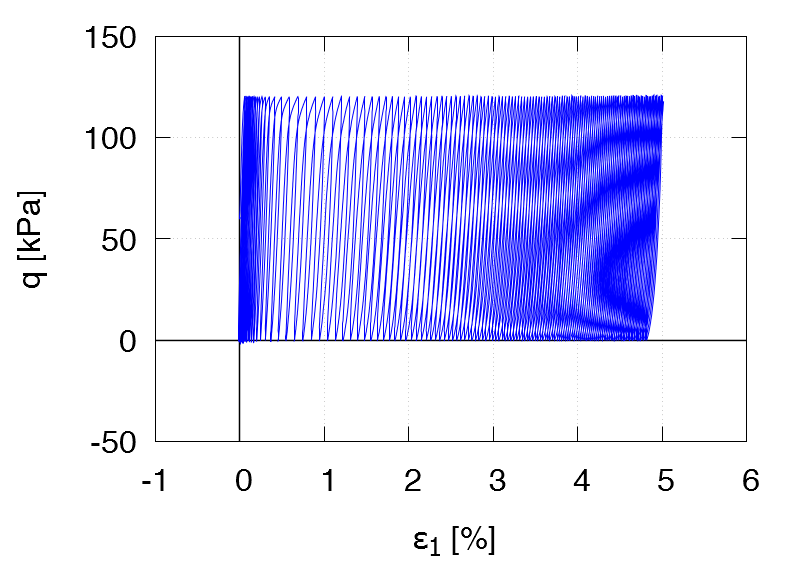TCUA16 ID0 = 0.56 p0 = 200 kPa η0 = 0.25 qampl = 60 kPa 1st drained cycle Test data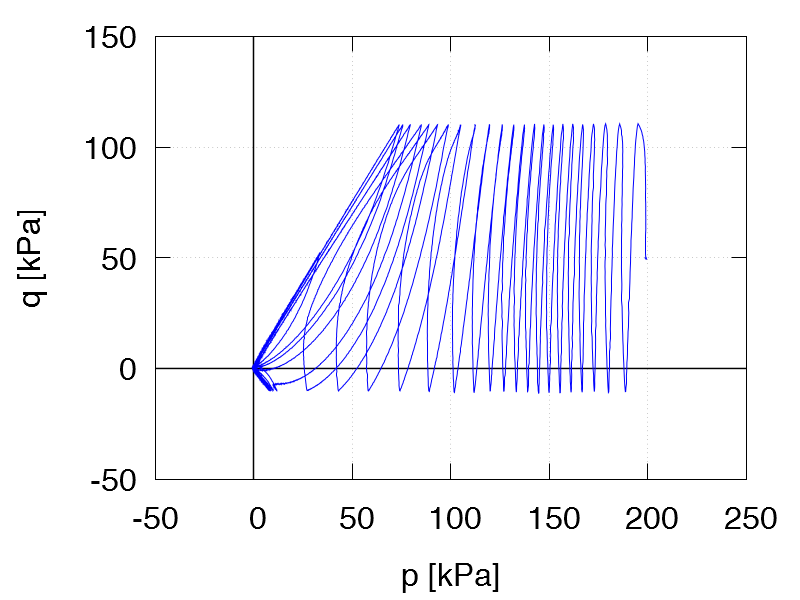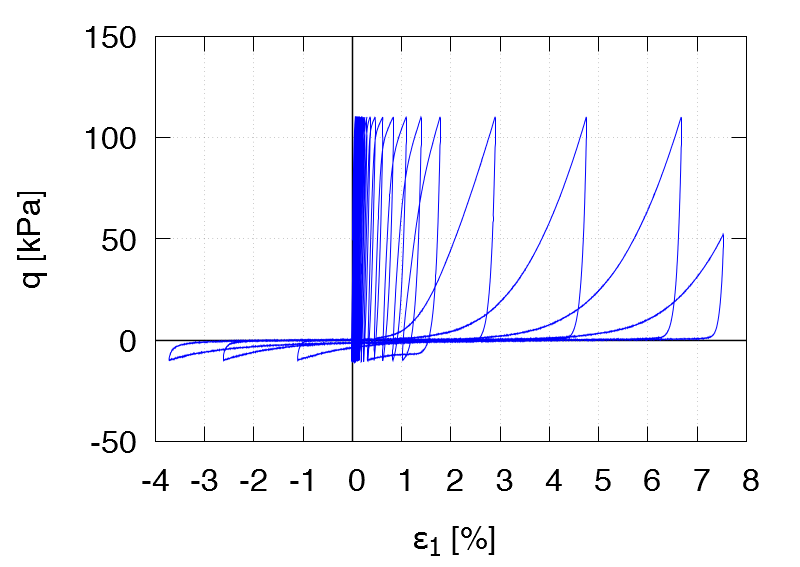TCUA17 ID0 = 0.65 p0 = 200 kPa η0 = -0.25 qampl = 60 kPa 1st drained cycle Test data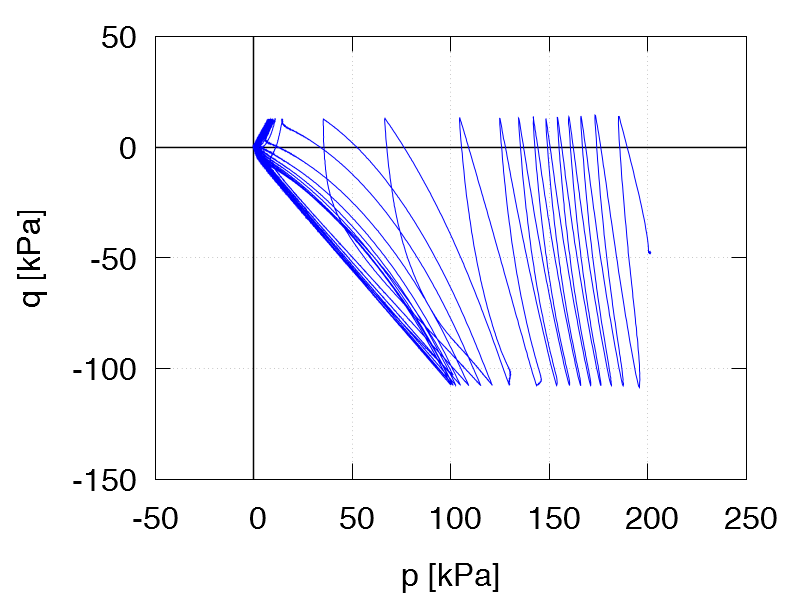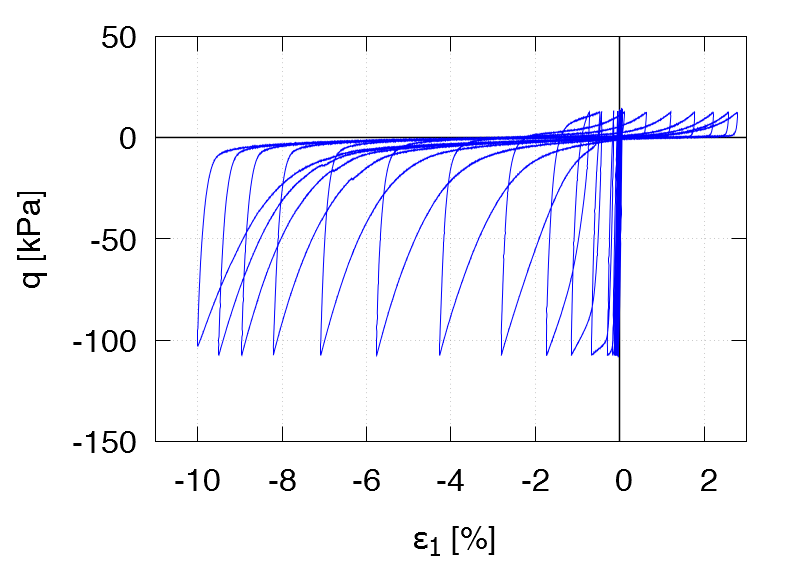TCUA18 ID0 = 0.61 p0 = 200 kPa η0 = -0.25 qampl = 40 kPa 1st drained cycle Test data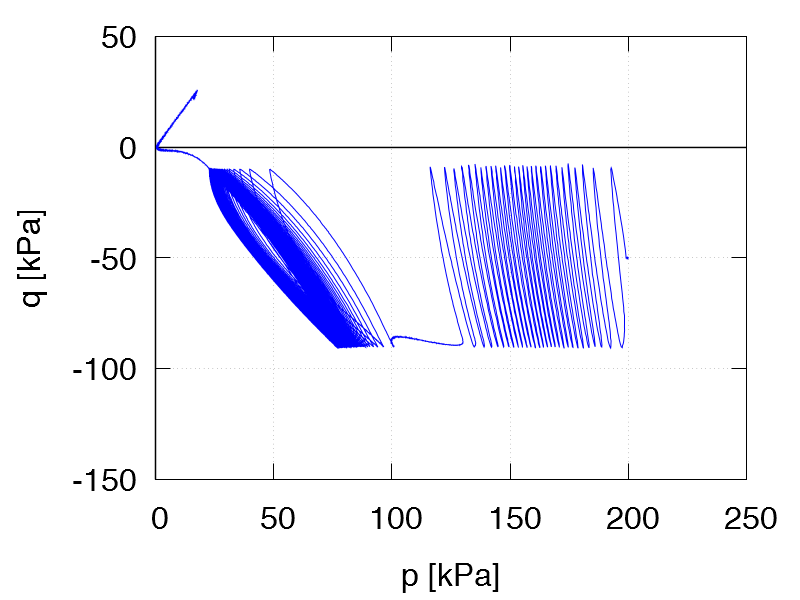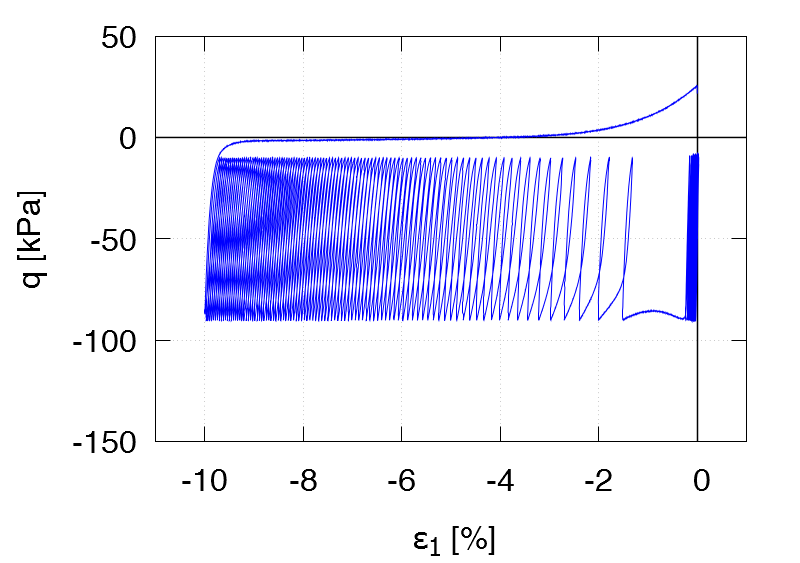TCUA19 ID0 = 0.66 p0 = 200 kPa η0 = -0.5 qampl = 40 kPa 1st drained cycle Test data